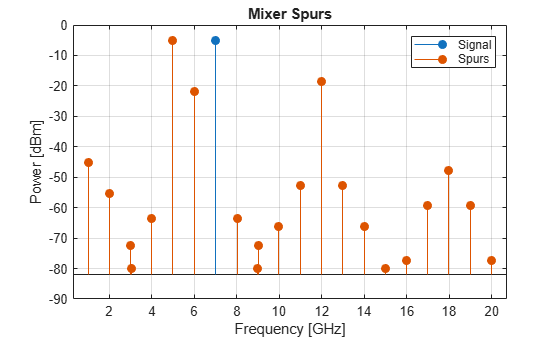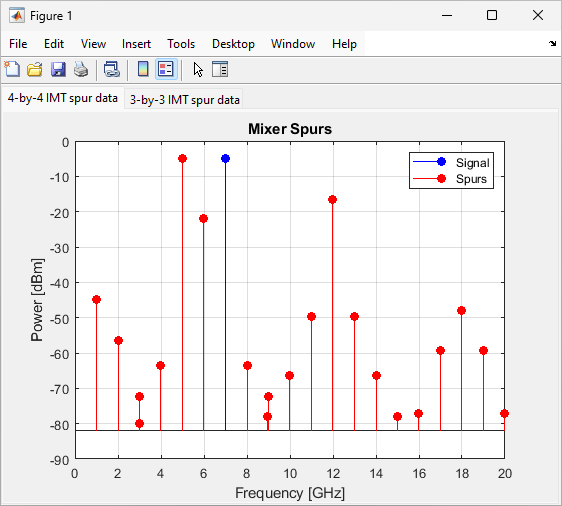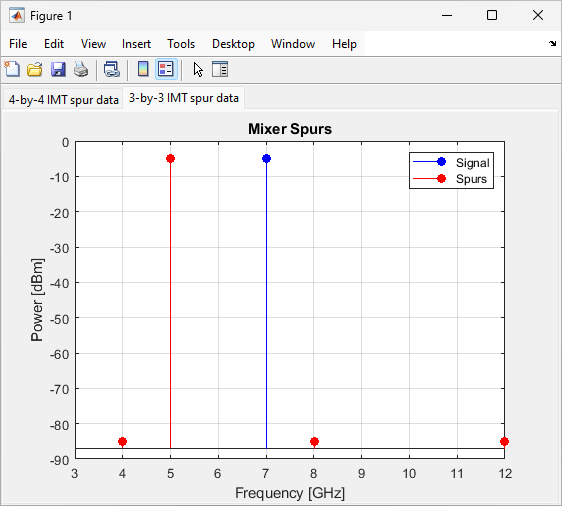# rfplot

Plot mixer spurs of IMT mixer

Since R2022a

## Syntax

``rfplot(imt,frequency)``
``rfplot(ax,imt,frequency)``

## Description

example

````rfplot(imt,frequency)` plots the mixer spurs response of the IMT mixer object `imt` at the frequency specified in `frequency`. The function plots the mixer spurs on the current axes, with the frequency in Hz on the X-axis and the input power in dBm on the Y-axis.```

example

````rfplot(ax,imt,frequency)` plots the mixer spurs on the axes specified in `ax` instead of the current axes. Return the current axes using the `gca` function.```

## Examples

collapse all

Create an IMT mixer with the LO frequency of 5995 MHz and with the IMT spurs.

```imt = mixerIMT(LO=5995e6,IMT=... [99 17 13.6 42.9; 40 0 47.7 54.3; 50.4 58.5 61.3 72.2; 75 67.4 75 99]);```

Plot the mixer spur at an input signal frequency of 1005 MHz.

`rfplot(imt,1005e6)`Create an IMT mixer with 4-by-4 IMT spur data.

```imt = mixerIMT(LO=5995e6,IMT=... [99 17 11.6 42.9; 40 0 44.7 54.3; 51.4 58.5 61.3 72.2; 75 67.4 73 99]);```

Create an IMT mixer with 3-by-3 IMT spur data.

```imt2 = mixerIMT(LO=5995e6,IMT=... [99 99 80; 99 0 99; 99 80 99]);```

Type these commands at the command line to create a `rfplot` figure with two tabs. Add axes to each tab by specifying the parent container for each axis. Plot the IMT mixer with the 4-by-4 IMT spur data in the first tab and the IMT mixer with the 3-by-3 IMT spur data in the second tab.

```figure tab1 = uitab('Title','4-by-4 IMT spur data'); ax3 = axes(tab1); rfplot(ax3,imt,frequency=1005e6, powerinput=-5) tab2 = uitab('Title','3-by-3 IMT spur data'); ax4 = axes(tab2); rfplot(ax4,imt2,frequency=1005e6, powerinput=-5) ```## Input Arguments

collapse all

IMT mixer object, specified as a `mixerIMT` object.

Data Types: `object`

Input frequency of the IMT mixer object, specified as a positive scalar in Hz.

Data Types: `double`

Axes to plot mixer spurs, specified as an `axes` object.

## Tips

In addition to plotting the mixer spurs, you can also use the `rfplot` function to:

## Version History

Introduced in R2022a